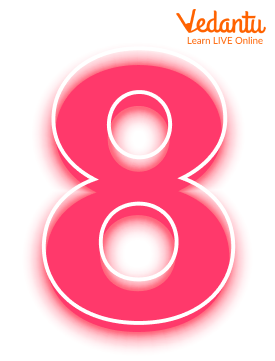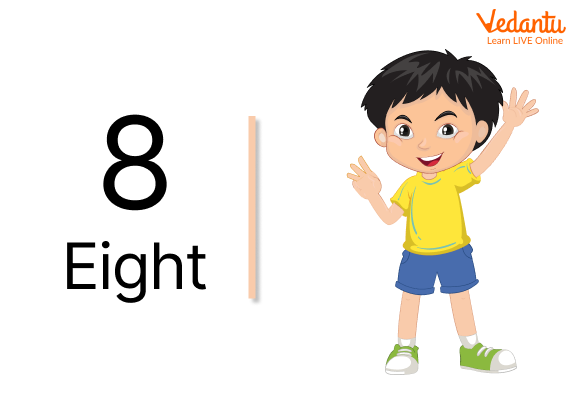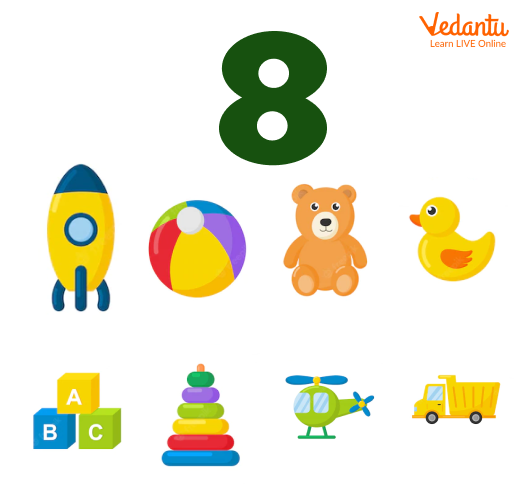Courses
Courses for Kids
Free study material
Free LIVE classes
More

# Number 8 in Maths: Easy Explanation for Kids## What is 8?

The number 8 (eight) is represented by a number and numeral. It's the natural number that comes after 7 and before 9. It's an integer and a cardinal number, which means it's a number that can be counted. It's also classified as a real number, which distinguishes it from imaginary numbers.

Let us understand what the number 8 means and how it is used in various categories from this section.

### What is 8 in Mathematics?

Eight is a composite number with the divisors 1, 2, and 4 as its appropriate divisors. Eight can be written as 23, totaling 23, which can be pronounced as two cubed. It has a sum of 7 aliquots. The aliquot sum of all powers of two is one less than their own.The Number 8

The octal number system, which is commonly used with computers, has an 8 as its basis. One digit in octal represents three bits. A byte, often known as an octet in modern computers, is a collection of eight bits.

The number 8 is a Fibonacci number, as it is made up of the numbers 3 and 5.

Under the second definition, where repeating prime elements are counted as often as they occur, 8 and 9 create a Ruth-Aaron pair.

An octagon is a polygon with eight sides. Octagonal numbers are figurate numbers that depict octagons (including eight). An octahedron is a polyhedron having eight faces.

### Examples on 8

Let us look at a few descriptions that represent the number 8 in this section.A little one showing his 8 fingersA set of 8 objects appeared at a place

### Interesting Facts

• Since 8 is a Fibonacci number, it can be found naturally all around the world. The number eight, the sixth in the Fibonacci sequence, is the only number in the sequence (apart from one) that is a perfect cube (23). The Fibonacci series continues with thirteen, while the previous number is five.

• An octagon is a two-dimensional object with eight sides, while an octahedron is a three-dimensional shape with eight faces. They are named from the prefix ‘oct’, which is used to signify the number eight in numerous English terms, including octopus (which has eight arms).

• A popular British confection is designed to be consumed in the evening, specifically after 8 p.m. The minty heart of Nestlé's After Eight Mint Chocolate Thins (or just After Eight) is covered with chocolate. Every year, more than a billion After Eights are sold around the world.

### Conclusion

Most of the children are much interested to represent the single digit using their fingers. And the number 8 is one of them. When parents or teacher asked children to show something that represents the number 8, they can immediately point their 8 fingers. That’s a good way of immediately responding and we can come to know that they have understood the topic. This is one of the good ways to teach children about the importance and working with the numbers.

Last updated date: 01st Oct 2023
Total views: 139.5k
Views today: 3.39k

## FAQs on Number 8 in Maths: Easy Explanation for Kids

1. How can we represent 8 in various categories?

Number 8 can be represented in various forms. A few of them are:

• 8 can often be used in the context of sports, such as skating.
• A fallen or lying down 8 is used to represent infinity (∞) in mathematics.

2. How to make your children learn the number 8?

Children can be asked to count or observe things in their environment that are eight in number. For example, you could talk about the number of pints in a gallon, the number of legs on a spider or scorpion, or the number of oars on the racing boat 'eight.'

Instead of giving children numerical worksheets, show them an atlas and ask them to count how many planets there are in our solar system.